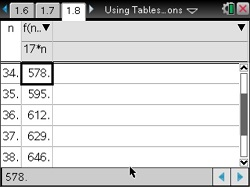••• ##### Device
•TI-Nspire™ CX/CX II
•TI-Nspire™ CX CAS/CX II CAS
• TI-Nspire™
• TI-Nspire™ CAS
• ##### Software

TI-Nspire™
TI-Nspire™ CAS

3.0

• ##### Report an Issue

Algebra 1: Using Tables to Solve Linear Equations
by Brendan Kelly#### Objectives

• Solve "one-step" linear equations of the form x + a = b and ax = b where a and b are real numbers
• Solve "two-step" linear equations of the form ax + b = c where a and b are real numbers